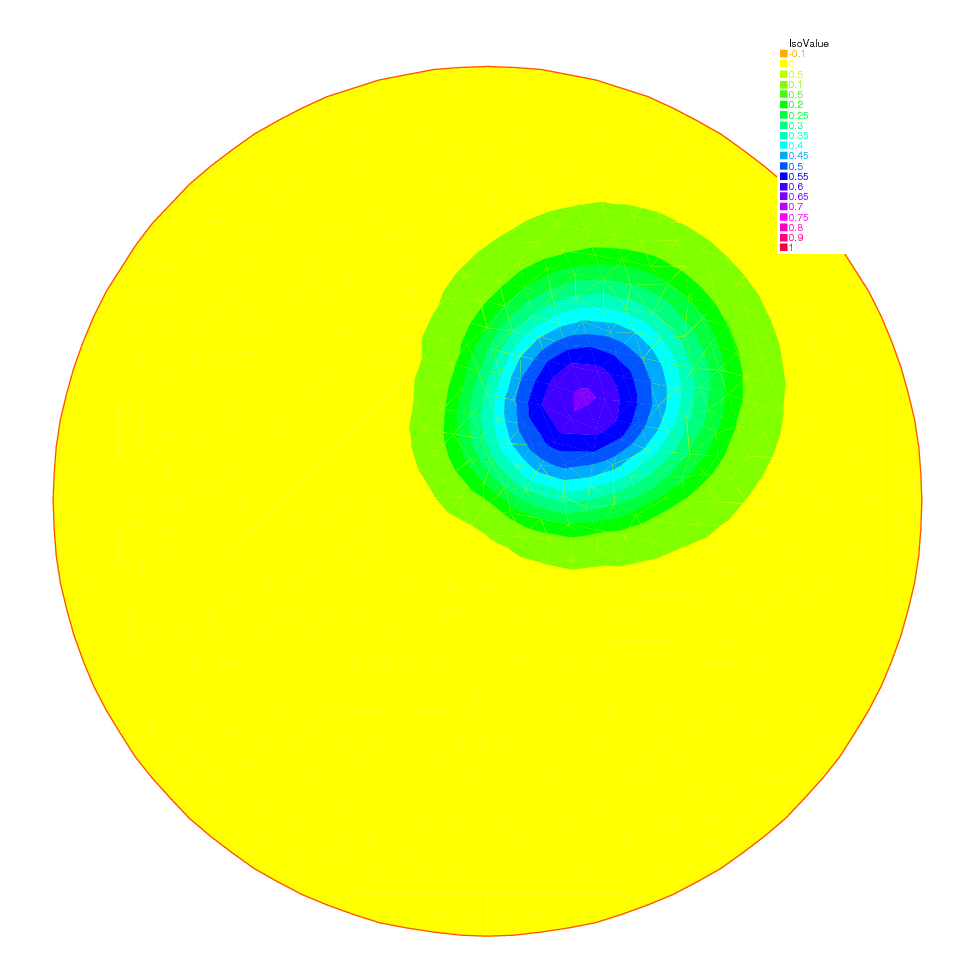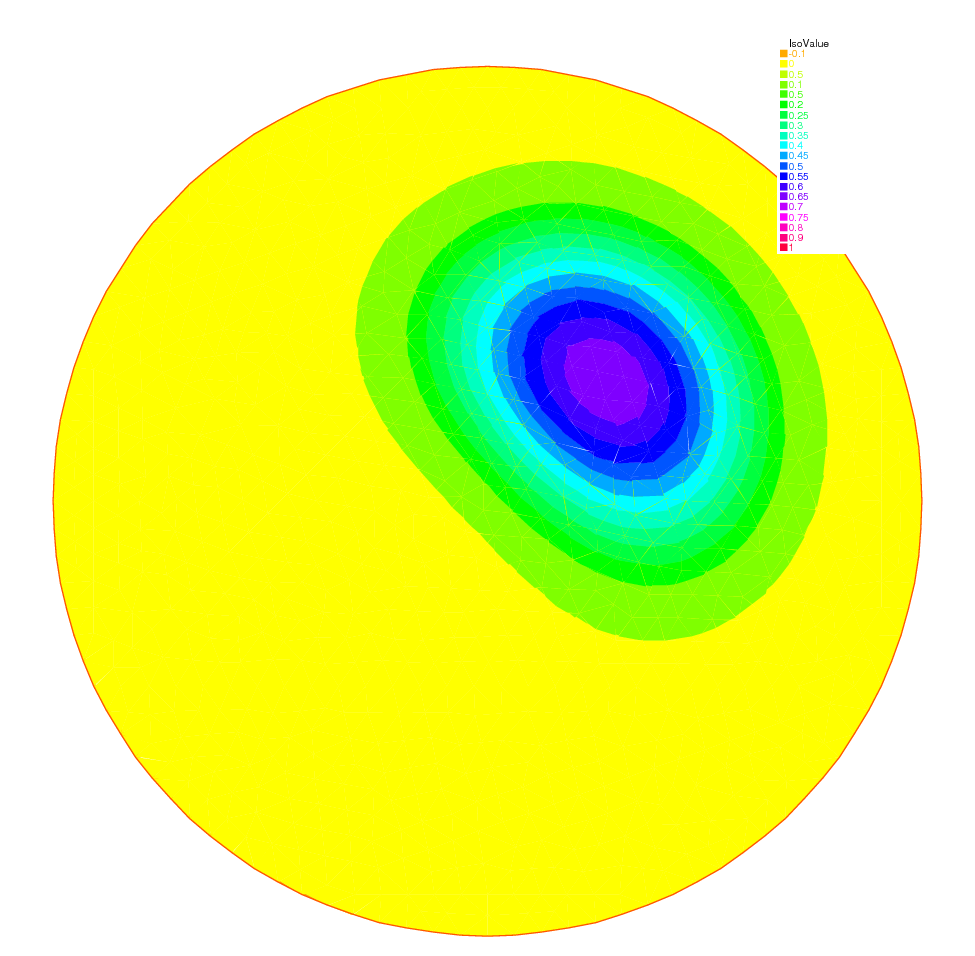FreeFEM Documentation on GitHub

stars - forks

# Pure Convection : The Rotating Hill

Summary: Here we will present two methods for upwinding for the simplest convection problem. We will learn about Characteristics-Galerkin and Discontinuous-Galerkin Finite Element Methods.

Let $$\Omega$$ be the unit disk centered at $$(0,0)$$; consider the rotation vector field

$\mathbf{u} = [u1,u2], \qquad u_1 = y,\quad u_2 = -x$

Pure convection by $$\mathbf{u}$$ is

$\begin{split}\begin{array}{rcl} \partial_t c + \mathbf{u}.\nabla c &= 0 &\hbox{ in } \Omega\times(0,T)\\ c (t=0) &= c ^0 &\hbox{ in } \Omega. \end{array}\end{split}$

The exact solution $$c(x_t,t)$$ at time $$t$$ en point $$x_t$$ is given by:

$c(x_t,t)=c^0(x,0)$

where $$x_t$$ is the particle path in the flow starting at point $$x$$ at time $$0$$. So $$x_t$$ are solutions of

$\dot{x_t} = u(x_t), \quad\ x_{t=0} =x , \quad\mbox{where}\quad \dot{x_t} = \frac{\text{d} ( x_t }{\text{d} t}$

The ODE are reversible and we want the solution at point $$x$$ at time $$t$$ ( not at point $$x_t$$) the initial point is $$x_{-t}$$, and we have

$c(x,t)=c^0(x_{-t},0)$

The game consists in solving the equation until $$T=2\pi$$, that is for a full revolution and to compare the final solution with the initial one; they should be equal.

## Solution by a Characteristics-Galerkin Method

In FreeFEM there is an operator called convect([u1,u2], dt, c) which compute $$c\circ X$$ with $$X$$ is the convect field defined by $$X(x)= x_{dt}$$ and where $$x_\tau$$ is particule path in the steady state velocity field $$\mathbf{u}=[u1,u2]$$ starting at point $$x$$ at time $$\tau=0$$, so $$x_\tau$$ is solution of the following ODE:

$\dot{x}_\tau = u(x_\tau), {x}_{\tau=0}=x.$

When $$\mathbf{u}$$ is piecewise constant; this is possible because $$x_\tau$$ is then a polygonal curve which can be computed exactly and the solution exists always when $$\mathbf{u}$$ is divergence free; convect returns $$c(x_{df})=C\circ X$$.

 1// Parameters
2real dt = 0.17;
3
4// Mesh
5border C(t=0., 2.*pi) {x=cos(t); y=sin(t);};
6mesh Th = buildmesh(C(100));
7
8// Fespace
9fespace Uh(Th, P1);
10Uh cold, c = exp(-10*((x-0.3)^2 +(y-0.3)^2));
11Uh u1 = y, u2 = -x;
12
13// Time loop
14real t = 0;
15for (int m = 0; m < 2.*pi/dt; m++){
16    t += dt;
17    cold = c;
18    c = convect([u1, u2], -dt, cold);
19    plot(c, cmm=" t="+t +", min="+c[].min+", max="+c[].max);
20}


Note

3D plots can be done by adding the qualifyer dim=3 to the plot instruction.

The method is very powerful but has two limitations:

• it is not conservative

• it may diverge in rare cases when $$|\mathbf{u}|$$ is too small due to quadrature error.

## Solution by Discontinuous-Galerkin FEM

Discontinuous Galerkin methods take advantage of the discontinuities of $$c$$ at the edges to build upwinding. There are may formulations possible. We shall implement here the so-called dual-$$P_1^{DC}$$ formulation (see [ERN2006]):

$\int_\Omega(\frac{c^{n+1}-c^n}{\delta t} +u\cdot\nabla c)w +\int_E(\alpha|n\cdot u|-\frac 12 n\cdot u)[c]w =\int_{E_\Gamma^-}|n\cdot u| cw~~~\forall w$

where $$E$$ is the set of inner edges and $$E_\Gamma^-$$ is the set of boundary edges where $$u\cdot n<0$$ (in our case there is no such edges). Finally $$[c]$$ is the jump of $$c$$ across an edge with the convention that $$c^+$$ refers to the value on the right of the oriented edge.

 1// Parameters
2real al=0.5;
3real dt = 0.05;
4
5// Mesh
6border C(t=0., 2.*pi) {x=cos(t); y=sin(t);};
7mesh Th = buildmesh(C(100));
8
9// Fespace
10fespace Vh(Th,P1dc);
11Vh w, ccold, v1 = y, v2 = -x, cc = exp(-10*((x-0.3)^2 +(y-0.3)^2));
12
13// Macro
14macro n() (N.x*v1 + N.y*v2) // Macro without parameter
15
16// Problem
18    = int2d(Th)(
19          (cc/dt+(v1*dx(cc)+v2*dy(cc)))*w
20    )
21    + intalledges(Th)(
22          (1-nTonEdge)*w*(al*abs(n)-n/2)*jump(cc)
23    )
24    - int2d(Th)(
25          ccold*w/dt
26    )
27    ;
28
29// Time iterations
30for (real t = 0.; t < 2.*pi; t += dt){
31    ccold = cc;
33    plot(cc, fill=1, cmm="t="+t+", min="+cc[].min+", max="+ cc[].max);
34}
35
36// Plot
37real [int] viso = [-0.2, -0.1, 0., 0.1, 0.2, 0.3, 0.4, 0.5, 0.6, 0.7, 0.8, 0.9, 1., 1.1];
38plot(cc, wait=1, fill=1, ps="ConvectCG.eps", viso=viso);
39plot(cc, wait=1, fill=1, ps="ConvectDG.eps", viso=viso);


Note

New keywords: intalledges to integrate on all edges of all triangles

$\mathtt{intalledges}(\mathtt{Th}) \equiv \sum_{T\in\mathtt{Th}}\int_{\partial T }$

(so all internal edges are see two times), nTonEdge which is one if the triangle has a boundary edge and two otherwise, jump to implement $$[c]$$.

Results of both methods are shown on Fig. 17 nad Fig. 18 with identical levels for the level line; this is done with the plot-modifier viso.

Notice also the macro where the parameter $$\mathbf{u}$$ is not used (but the syntax needs one) and which ends with a //; it simply replaces the name n by (N.x*v1+N.y*v2). As easily guessed N.x,N.y is the normal to the edge.Fig. 17 The rotating hill after one revolution with Characteristics-GalerkinFig. 18 The rotating hill after one revolution with Discontinuous $$P_1$$ Galerkin

Rotating hill

Now if you think that DG is too slow try this:

 1// Mesh
2border C(t=0., 2.*pi) {x=cos(t); y=sin(t);};
3mesh Th = buildmesh(C(100));
4
5fespace Vh(Th,P1);//P1,P2,P0,P1dc,P2dc, uncond stable
6
7Vh vh,vo,u1 = y, u2 = -x, v = exp(-10*((x-0.3)^2 +(y-0.3)^2));
8real dt = 0.03,t=0, tmax=2*pi, al=0.5, alp=200;
9
10problem  A(v,vh) = int2d(Th)(v*vh/dt-v*(u1*dx(vh)+u2*dy(vh)))
11  + intalledges(Th)(vh*(mean(v)*(N.x*u1+N.y*u2)
12                   +alp*jump(v)*abs(N.x*u1+N.y*u2)))
13  + int1d(Th,1)(((N.x*u1+N.y*u2)>0)*(N.x*u1+N.y*u2)*v*vh)
14  - int2d(Th)(vo*vh/dt);
15
17  + intalledges(Th)((1-nTonEdge)*vh*(al*abs(N.x*u1+N.y*u2)
18                             -(N.x*u1+N.y*u2)/2)*jump(v));
19
20varf rhs(vo,vh)= int2d(Th)(vo*vh/dt);
21
22real[int] viso=[-0.1,0,0.1,0.2,0.3,0.4,0.5,0.6,0.7,0.8,0.9,1,1.1];
23